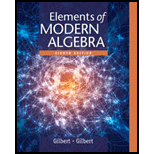# Let S be a set of three elements given by S = { A , B , C } . In the following table, all of the elements of S are listed in a row at the top and in a column at the left. The result x ∗ y is found in the row that starts with x at the left and in the column that has y at the top. For example, B ∗ C = C and C ∗ B = A . Thus the table defines the binary operation ∗ on the sets S . ∗ A B C A C A B B A B C C B A C a. Is the binary operation ∗ commutative? Why? b. Determine whether there is an identity element in S for ∗ . c. If there is an identity element, which elements have inverses?### Elements Of Modern Algebra

8th Edition
Gilbert + 2 others
Publisher: Cengage Learning,
ISBN: 9781285463230### Elements Of Modern Algebra

8th Edition
Gilbert + 2 others
Publisher: Cengage Learning,
ISBN: 9781285463230

#### Solutions

Chapter
Section
Chapter 1.4, Problem 3E
Textbook Problem

## Expert Solution

### Want to see the full answer?

Check out a sample textbook solution.See solution

### Want to see this answer and more?

Experts are waiting 24/7 to provide step-by-step solutions in as fast as 30 minutes!*

See Solution

*Response times vary by subject and question complexity. Median response time is 34 minutes and may be longer for new subjects.